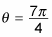##### Pre-Calculus For Dummies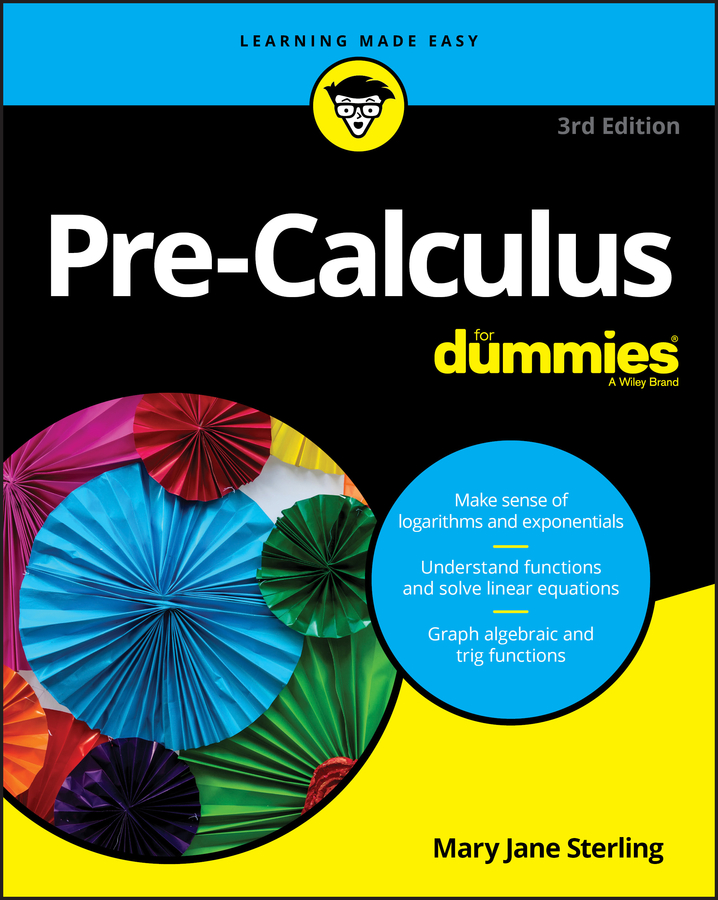Knowing how to calculate the circumference of a circle and, in turn, the length of an arc — a portion of the circumference — is important in pre-calculus because you can use that information to analyze the motion of an object moving in a circle.

An arc can come from a central angle, which is one whose vertex is located at the center of the circle. You can measure an arc in two different ways:

• As an angle. The measure of an arc as an angle is the same as the central angle that intercepts it.

• As a length. The length of an arc is directly proportional to the circumference of the circle and is dependent on both the central angle and the radius of the circle.

If you think back to geometry, you may remember that the formula for the circumference of a circle is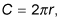with r representing the radius. Also recall that a circle has 360 degrees. So if you need to find the length of an arc, you need to figure out what part of the whole circumference (or what fraction) you're looking at.

You use the following formula to calculate the arc length: The symbol theta (θ) represents the measure of the angle in degrees, and s represents arc length, as shown in the figure: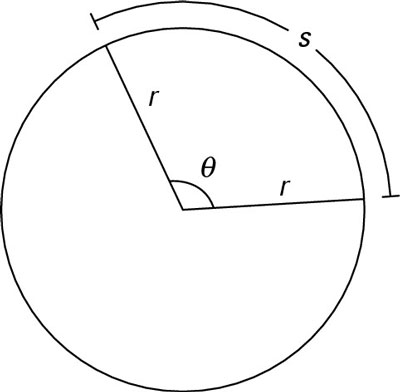The variables involved in computing arc length.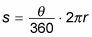If the given angle theta is in radians,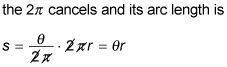Time for an example. To find the length of an arc with an angle measurement of 40 degrees if the circle has a radius of 10, use the following steps:

1. Assign variable names to the values in the problem.

The angle measurement here is 40 degrees, which is theta. The radius is 10, which is r.

2. Plug the known values into the formula.

This step gives you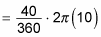3. Simplify to solve the formula.

You first get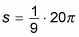which multiplies to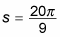The figure shows what this arc looks like.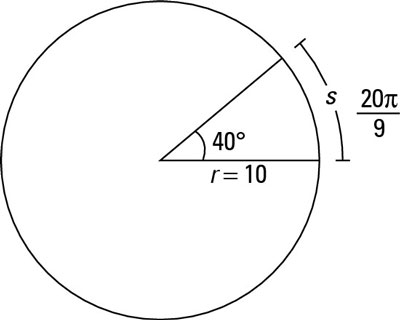The arc length for an angle measurement of 40 degrees

Now try a different problem. Find the measure of the central angle of a circle in radians with an arc length of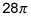and a radius of 16. This time, you must solve for theta (the formula is s = rθ when dealing with radians):

1. Plug in what you know to the radian formula.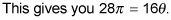2. Divide both sides by 16.

Your formula looks like this: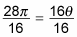3. Reduce the fraction.

You're left with the solution: